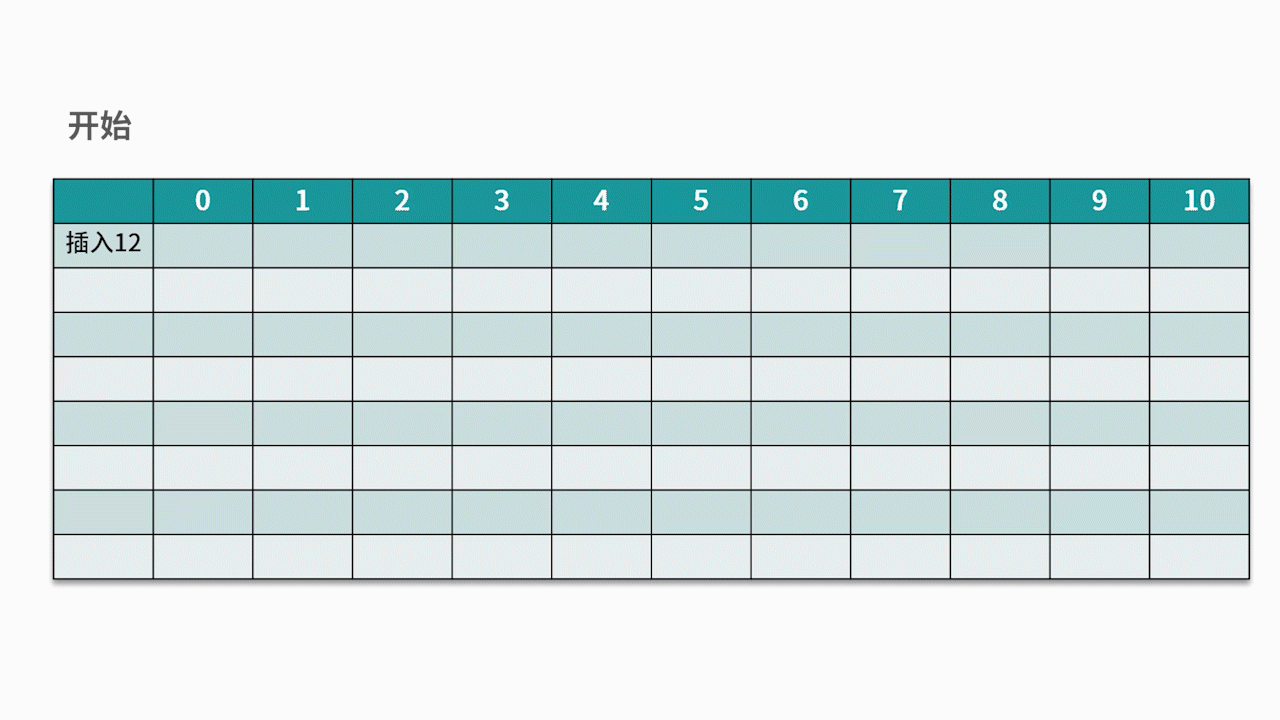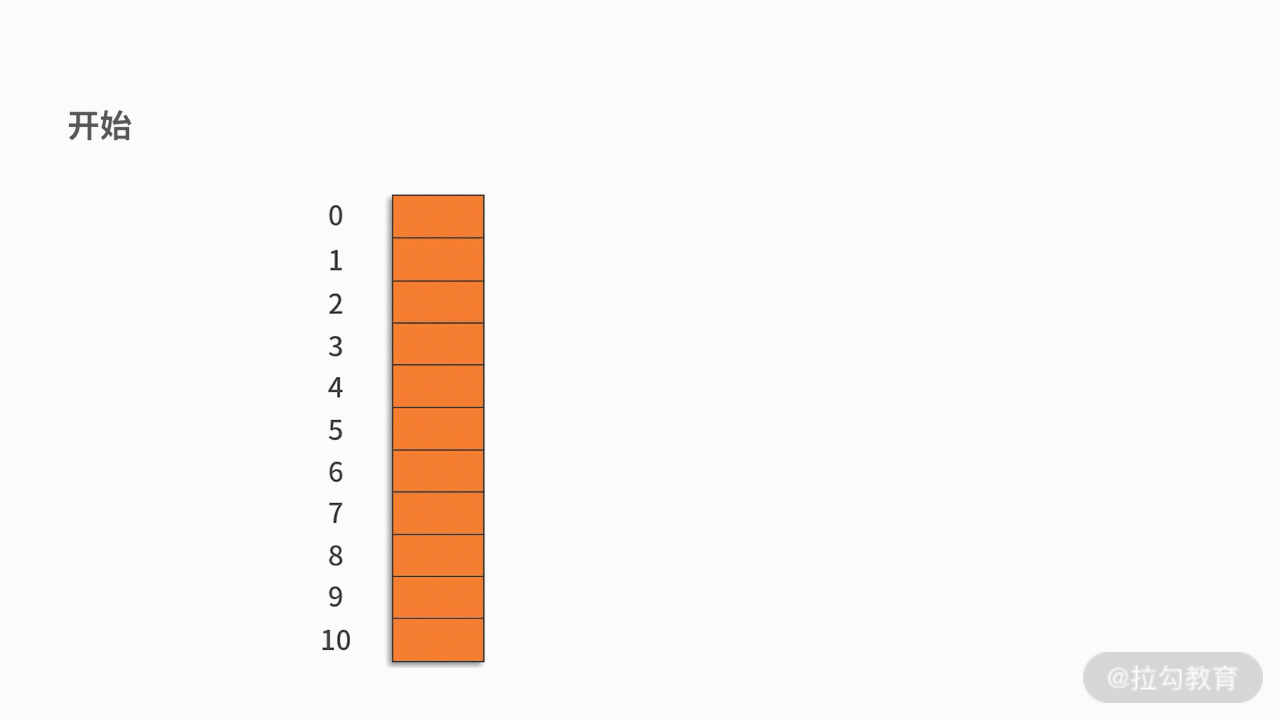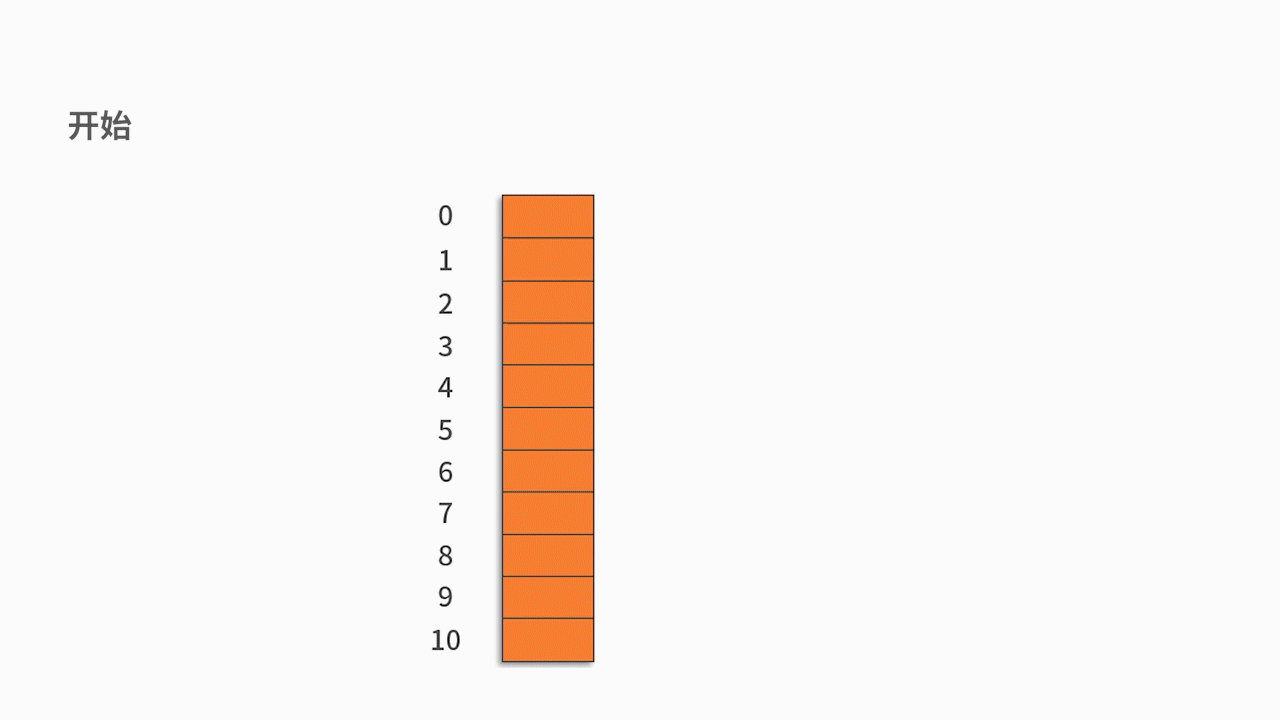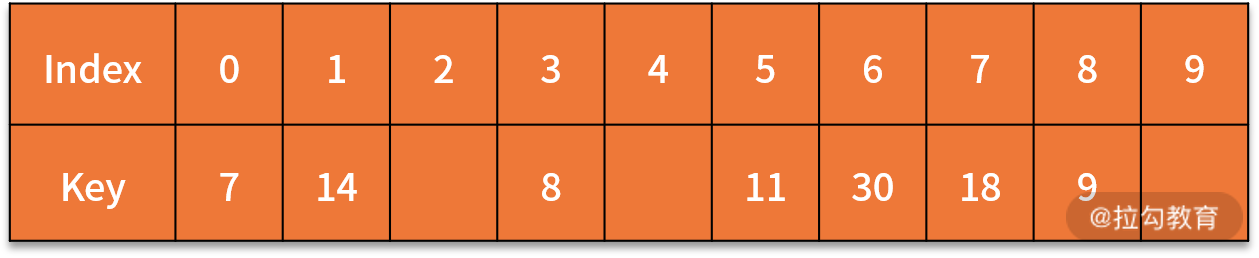10 哈希表：如何利用好高效率查找的“利器”？

• 线性表中的栈和队列对增删有严格要求，它们会更关注数据的顺序。
• 数组和字符串需要保持数据类型的统一，并且在基于索引的查找上会更有优势。
• 树的优势则体现在数据的层次结构上。

什么是哈希表

哈希表的核心思想

address (张一) = ASCII (Z) + ASCII (Y) = 90 + 89 = 179；

address (张二) = ASCII (Z) + ASCII (E) = 90 + 69 = 159；

address (张三) = ASCII (Z) + ASCII (S) = 90 + 83 = 173；

address (张四) = ASCII (Z) + ASCII (S) = 90 + 83 = 173；

• 第一，直接定制法

• 第二，数字分析法

• 第三，平方取中法

• 第四，折叠法

• 第五，除留余数法

如何解决哈希冲突

• 第一，开放定址法• 第二，链地址法哈希表的基本操作

• 如果哈希地址对应的值为空，则查找不成功。
• 反之，则查找成功。

哈希表的案例

H (7) = (7 * 3) % 7 = 0

H (8) = (8 * 3) % 7 = 3

H (30) = 6

H (11) = 5

H (18) = 5

H (9) = 6

H (14) = 0• 查找 7。输入 7，计算得到 H (7) = 0，根据哈希表，在 0 的位置，得到结果为 7，跟待匹配的关键字一样，则完成查找。
• 查找 18。输入 18，计算得到 H (18) = 5，根据哈希表，在 5 的位置，得到结果为 11，跟待匹配的关键字不一样（11 不等于 18）。因此，往后挪移一位，在 6 的位置，得到结果为 30，跟待匹配的关键字不一样（11 不等于 30）。因此，继续往后挪移一位，在 7 的位置，得到结果为 18，跟待匹配的关键字一样，完成查找。

• 如果存在，则把它对应的value（用来记录出现的频次）加 1；
• 如果不存在，则把它添加到 d 中，对应的 value 赋值为 1。最后，打印处 key_str 对应的 value，即累积出现的频次。

if (d.containsKey(key_str) {
d.put(key_str, d.get(key_str) + 1);
}
else{
d.put(key_str, 1);
}
System.out.println(d.get(key_str));